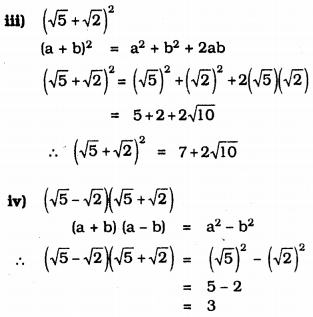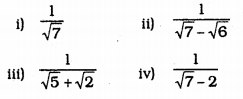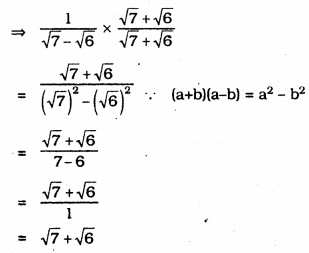# KSEEB Solutions for Class 9 Maths Chapter 1 Number Systems Ex 1.5

KSEEB Solutions for Class 9 Maths Chapter 1 Number Systems Ex 1.5 are part of KSEEB Solutions for Class 9 Maths. Here we have given Karnataka Board Class 9 Maths Chapter 1 Number Systems Exercise 1.5.

## Karnataka Board Class 9 Maths Chapter 1 Number Systems Ex 1.5

Question 1.
Classify the following numbers as rational or irrational.
i) $$2-\sqrt{5}$$
ii) $$(3+\sqrt{23})-\sqrt{23}$$
iii) $$\frac{2 \sqrt{7}}{7 \sqrt{7}}$$
iv) $$\frac{1}{\sqrt{2}}$$
v) 2π
i) $$2-\sqrt{5}$$ = 2 – 2.2360679……….. = -0.2360679
This is a non-terminating, non-recurring decimal.
∴ This is an irrational number.

ii) $$(3+\sqrt{23})-\sqrt{23}$$
= $$3+\sqrt{23}-\sqrt{23}$$
= 3
⇒ $$\frac{3}{1}$$. This can be written in the form of $$\frac{p}{q}$$.
∴ This is a rationl number.

iii) $$\frac{2 \sqrt{7}}{7 \sqrt{7}}=\frac{2 \sqrt{7}}{\sqrt{7}}=2 \Rightarrow \frac{2}{1}$$
This can be written in the form of $$\frac{p}{q}$$
∴ This is a rational number.

iv) $$\frac{1}{\sqrt{2}}$$
$$\frac{1}{\sqrt{2}} \times \frac{\sqrt{2}}{\sqrt{2}}=\frac{\sqrt{2}}{2}=\frac{1.4142}{2}$$
= 0.707106…………
This is a non-terminating, non-recurring decimal.
∴ This is an irrational number.

v) 2π
= 2 × 3.1415…..
= 6.2830…….
This is a non-terminating, non-recurring decimal.
∴ This is an irrational number.

Question 2.
Simplify each of the following expressions:
i) $$(3+\sqrt{3})(2+\sqrt{2})$$
ii) $$(3+\sqrt{3})(3-\sqrt{3})$$
iii) $$(\sqrt{5}+\sqrt{2})^{2}$$
iv) $$(\sqrt{5}-\sqrt{2})(\sqrt{5}+\sqrt{2})$$Question 3.
Recall, 2π is defined as the ratio of the circumference (say c) of a circle to its diameter (say d). That is, $$\pi=\frac{c}{d}$$. This seems to contradict the fact that n is irrational. How will you resolve this contradiction?
There is no contradiction. When we measure a length with a scale or any other device we get the quotient.
Therefore we cannot judge whether c is a rational number of d is an irrational number.
∴ Value of $$\frac{c}{d}$$ is irrational number.
∴ The value of π is also an irrational number.

Question 4.
Represent $$\sqrt{9.3}$$ on the number line.
Construction: Mark the distance 9.5 units from a fixed point O such that OB = 9.3 units. Mark midpoint D of OC. Draw a semicircle with centre D. Draw a line perpendicular to OC passing through E and intersecting the semicircle at E. Draw an arc BE which intersect at F. Now, BF = $$\sqrt{9.3}$$.Question 5.
Rationalise the denominators of the following :i) $$\frac{1}{\sqrt{7}}$$
Denominator’s factor is $$\sqrt{7}$$ Mulitplying Numerator and denominator by $$\sqrt{7}$$.ii) $$\frac{1}{\sqrt{7}-\sqrt{6}}$$
Denominator’s factor is $$\sqrt{7}+\sqrt{6}$$
Multiplying numerator and denominator by $$\sqrt{7}+\sqrt{6}$$,iii) $$\frac{1}{\sqrt{5}+\sqrt{2}}$$
Denominator’s factor is $$\sqrt{5}-\sqrt{2}$$
Multiplying numerator and denominator by $$\sqrt{5}-\sqrt{2}$$.iv) $$\frac{1}{\sqrt{7}-2}$$
Denominator’s factor is $$\sqrt{7}+2$$
Multiplying numerator and denominator by $$\sqrt{7}+2$$.We hope the KSEEB Solutions for Class 9 Maths Chapter 1 Number Systems Ex 1.5 help you. If you have any query regarding Karnataka Board Class 9 Maths Chapter 1 Number Systems Exercise 1.5, drop a comment below and we will get back to you at the earliest.

error: Content is protected !!